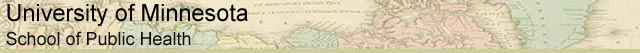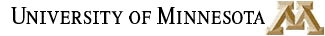### MATH REVIEW: USEFUL MATH FOR EVERYONE

 Ratios and Proportions Algebraic Expressions Exponents Logarithms Glossary and Links

denominator
the bottom number in a fraction; i.e. in the fraction 19/32, 32 is the denominator.

exponent
the number of times that a given number, such as n, is multiplied by itself; i.e. n to the third power, or n3, means n x n x n

fraction
when the units of a ratio are the same, a ratio may be written as a fraction. Two apples to three oranges (2:3) could not be written as a fraction. The ratio of two apples to three apples may be written in two ways: with a colon 2:3 or as a common fraction 2/3. The fraction 2/3 may also be interpreted as 2 divided by 3. A common fraction has two parts: the numerator and the denominator. In the fraction 2/3, 2 is the numerator and 3 is the denominator.

logarithm
a logarithm is the “power” to which a number must be raised in order to “get” some other number. The two most common logarithms are base 10 logarithms (the base unit is the number being “raised to a power”) and natural logarithms.

logarithm, base ten
a base ten logarithm is the exponent of 10 required for the expression to equal some other number. It is usually written in this form: log a=r, which means that a=10^r.

logarithm, natural
a natural logarithm is the exponent of e ~ 2.71828183 required for the expression to equal some other number. e is a transcendential number, which means it does not end. It is usually written in this form: ln a=r, which means that a=e^r.

numerator
the top number in a fraction; i.e.in the fraction 19/32, 19 is the numerator.

proportion
a proportion is a statement that expresses two equal ratios. A ratio is different from a proportion.

ratio
a ratio is a relationship involving a comparison of two numbers in a definite order, such as "two apples to three oranges." Ratios are usually written in this form: 2:3. When the units of both numbers in a ratio are the same, the ratio may be written as a fraction.